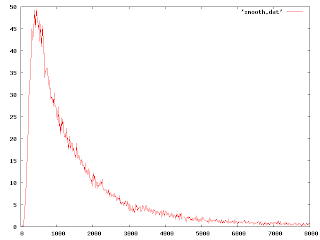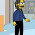## Thursday, July 2, 2009

### Too much information

I won't keep you in suspense. Here is my time series after removing the hair:I sliced up the curve into approximate 15 ms segments. I offset the segments so that the spike would be about in the middle, then I simply averaged each segment independently and pasted the result back together. This does remove details smaller than about 15 ms, but I don't need that level of detail.

Actually the whole point of what I'm doing is to remove detail. The original time series had way too much. I want to remove as much detail as possible, but keep just enough so I can easily characterize this data set and compare it with others. At one end of the detail scale I have the original data set of 8192 values. The other end of the scale has no data whatsoever (trivial, but very parsimonious). A number at least allows some basis of comparison. But which number? If I told you that the average value was about 1572 ms, would that be useful? Well, that depends. You might be mislead to expect that if you were to try the experiment right now that it would take around 1572 ms, more or less. You'd be wrong. More than 65% — almost two thirds — of the samples are below the average. The average is the total amount of time to gather all the samples divided by the number of samples gathered. This tells you a lot about the rate at which you can expect to gather samples in bulk, but not very much about the time value of an individual sample.

We need a parameterized model for our data set. The model will tell us qualitatively what the data set is like, the parameters will give us the quantitative information we need to compare a particular data set against others. If you don't know what the model is, the numbers are meaningless! This is no exaggeration. In this chart, there are two distributions. Both distributions have the same average.Whenever I see a measure of “average latency” a little alarm bell goes off in my head. It's a good indication that the stated value is a meaningless number and that the person who measured it doesn't know what he is measuring. A louder alarm bell goes off when I see a measure of “standard deviation”. Informally, the standard deviation is a measure of how widely the data is distributed. But the way you calculate the standard deviation depends on the model. (The relevance of the standard deviation also depends on the model. If your model is logarithmic, as most time-based models are, you would be better off computing the ‘geometric standard deviation’.) If the model is not specified, it is likely that the reported ‘standard deviation’ was simply calculated with a gaussian model. Some models don't even have a standard deviation — it's simply not defined.

When I see a measure of standard deviation, it's a good indication that the person who measured it not only doesn't know what he is measuring, but also that he is using a tool that he doesn't understand.

In order to figure out how to characterize my data set, I need to find a good model for it. This can be really hard. There are hundreds of models. Some of them have enough tuning parameters that you can make them fit anything. What I'm looking for is a model that is simple, has as few parameters as possible, and fits the data reasonably well. (The reason I shaved the hair off the data is so I could visually compare the fit of a few different models.) There are a number of ways to search for models, but it often comes down to trial and error. There are a couple of tricks, though.

The first trick is see how the data looks in log space. It is frequently the case that you are working with some ‘scale-free’ quantity. You don't care about the absolute time, you care about the relative improvement. When you discuss data in terms of ‘percent’ or ‘factor of two’ and such, you are likely to want to work in log space. Here's a plot of my shaved data set in log space:Now that is starting to look like a bell-shaped curve. This suggests that a log-normal distribution might be a good model:And now I can state quantitatively that under a log-normal model, my data set has a value of μ= 6.97 and σ=.90 With just these two numbers, you can approximate the mean (the true average is 1572, the average of the model is 1593), the mode (472), and any other quantity of the curve (for example, the geometric standard deviation, which is 2.46).

But the log-normal curve doesn't quite fit. It's close, but it overestimates near the mean and underestimates the long tail. I've been looking for a better model.

Next time.... trying different models.

1.I'm really enjoying the series (no pun intended). Thanks Joe.

2.It looks like a Weibull distribution to me.

Why not tell us what you're measuring? You gave us some clues: it's a time series and it's affected by Windows' CPU scheduler. I'd guess it's a queue length or response time measurement, but I haven't thought of a phenomenon that would give that big hump.

3.I can't yet give details about the source, but I may be able to in the future.